## Using Operators in Swift

What You’ll Learn in This Hour:

• How to identify unary, binary, and ternary operators
• How to use arithmetic operators
• How to use logical operators
• How to use assignment and equality operators
• How to use operators with strings
• How to use range operators

Operators are a key ingredient to every app. In fact, it’s difficult to write an app—other than the standard “Hello, world!” example—that doesn’t contain at least one operator. Operators are symbols or a set of symbols that change or assign values, combine values, or check or verify values in your code. In this hour, we learn about most of the basic operators in Swift and understand why and how to use them.

All operators in Swift can be categorized as unary, binary, or ternary. Unary operators perform an action upon a single operand, binary operators perform an action upon two operands, and ternary operators perform an action involving three operands.

### Unary Operators

Video 3.1—Use Unary Operators

A unary operator operates on a single value. A unary operator can be prefix or postfix, meaning that it can come before a variable or constant (prefix, such as `++count`), or immediately follow a variable or constant (postfix, such as `count++`). Some unary operators can be either (prefix or postfix), while some can be only one or the other. A unary operator cannot have any whitespace between itself and the variable or constant. Unary operators act upon numeric and Boolean types in Swift. Let’s take a look at Swift’s unary operators.

#### Increment and Decrement Operators

Two similar operators are the increment operator and the decrement operator. The increment operator, denoted by `++`, increases a numeric value by 1, and the decrement operator, denoted by `--`, decreases a numeric value by 1. The increment operator is short-hand for a longer expression; `a++` is the same as `a = a + 1`, and `a--` is the same as `a = a - 1`. Both the increment and decrement operators can be prefix or postfix.

There is a key difference in behavior, however, between the increment and decrement operators concerning prefix and postfix. That is the order in which the value is incremented or decremented and when assignment occurs. A prefixed decrement operator decrements the numeric value by 1 and returns the newly assigned value. Likewise, a prefixed increment operator increments the numeric value by 1 and returns the newly assigned value. The postfixed decrement operator first returns the numeric value before decrementing; likewise, the postfixed increment operator returns the numeric value before incrementing. Take a look at the following code example to see this behavior in action:

`var x = 1print(++x)//++x - x increments first, new value is displayedx = 1//just resetting value for demonstrationprint(x++)//now x is incremented, but after the print function has already occurredprint(x)//notice the different value now when this line is printed?`

#### Logical NOT Operator

The logical NOT operator inverts the value of a Boolean variable. In other words, if a variable’s value is `true`, the variable will now be `false`, and vice versa. The logical NOT operator is always a prefix operator. We discuss the logical NOT operator and other logical operators a little more in depth later in this hour. Here is what the logical NOT operator looks like in code:

`let a = true // a is a Bool equaling truelet b = !a // b is a Bool equaling false`

Caution: The Logical NOT Operator Must Be a Prefix Operator

Using (`!`) after a special type of variable, called an optional value, has another meaning, which is covered in Hour 6, “Understanding Optional Values.”

#### Unary Minus Operator

The unary minus operator is short-hand for multiplying a variable’s value by -1, and returning the result. The unary minus operator is always a prefix operator and can prefix variables, constants, or numeric literals. Here is what the unary minus operator looks like in code:

`let c = 5let d = -c // d is equal to -5let e = -42 // e is equal to -42`

Swift also has a unary plus operator, by prefixing a `+` before any variable or constant, but it doesn’t actually change the value of a numeric variable, because any negative multiplied by a positive is still a negative. The unary plus operator is more for distinction in your code to show that a certain value should be positive. It is very rarely used, but it is available.

### Binary Operators

Video 3.2—Use Binary Operators

Binary operators are operators that affect operands, which are the values on either side of the operator. For example, take `a + b`. In this expression, `a` and `b` are operands, and `+` is the operator. All Binary operators are infix, meaning they are in between two operands.

#### Standard Arithmetic Operators

Swift provides the four standard arithmetic operators that are binary operators for all numeric types, which are addition (+), subtraction (-), division (/), and multiplication (*). To use these operators in code, you use them exactly the way you would write them in a math equation:

`let a = 5 + 5 // a equals 10let b = 3 - 2 // b equals 1let c = 8.0 / 5.0 // c equals 1.6let d = 4 * 4 // d equals 16`

#### Remainder Operator

Swift includes a remainder operator, annotated by the `%` sign. In other languages, this operator is called the modulo operator, which has slightly different behavior. Swift calculates the remainder operator by figuring out how many times `b` evenly divides `a`, and returns the remainder. In other words, `a = (b * multiplier) + remainder`, with `multiplier` being the largest number `b` could multiply against to not produce a value higher than `a`.

In languages like C and Objective-C, the modulo operator behaved similarly, but unlike Swift, it could not perform against floating-point numbers; you would get a compiler error as the modulo operator cannot take floating-point numbers as its operands. This is another reason that Swift’s remainder operator is truly a remainder operator, because Swift gives the remainder of what is left over from `a - (b * multiplier)`. Listing 3.1 displays the modulo operator in C.

LISTING 3.1 Modulo Operator in C and Objective-C

`int a = 8;int b = 6;int c = a % b; // returns 2, as expectedint d = -8;int e = d % b; // returns -2, as expected`

In C and Objective-C, the modulo operator is pretty straightforward, as long as you’re providing integers. Listing 3.2 displays the remainder operator in Swift, which not only works with integers like C, but can also operate on `Double` types.

LISTING 3.2 Remainder Operator in Swift

`let a = 8let b = 6let c = a % b // returns 2, just as in Clet d = 8.0let e = 2.5let f = d % e // returns 0.5, Swift allows remainders for Double types`

#### The Assignment Operator

There is just one assignment operator in Swift, as in other languages, and that is the equal sign (`=`). The assignment operator takes the calculated or literal value on the right side of the equal sign and assigns it to the variable or constant on the left side of the equal sign. We have seen the assignment operator many times throughout this book already, and every single line in Listing 3.1 and Listing 3.2 utilizes the assignment operator.

#### Compound Assignment Operators

Compound assignment operators in Swift utilize the previously mentioned assignment operator (`=`), coupled with another operator, to perform a combined effect. Table 3.1 details each operator, what code it is short for, and a description.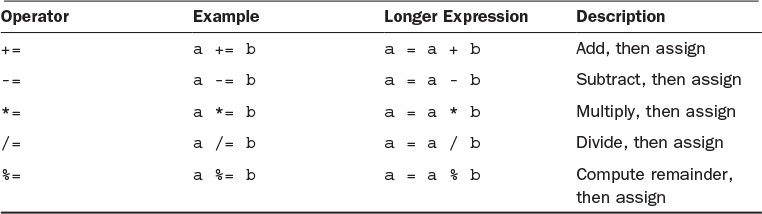TABLE 3.1 Compound Assignment Operators

Table 3.1 shows some of the basic compound operators. However, there are many more compound operators, all of which are more advanced than the scope of this book, such as bitwise and logical compound operations. For more information on these operators, see the chapter on expressions in The Swift Programming Language, Apple, 2015.

#### Comparison Operators

Comparison operators in Swift are similar to those of other programming languages. Each one returns a Boolean value describing whether the expression was true or false. Table 3.2 lists the basic comparison operators.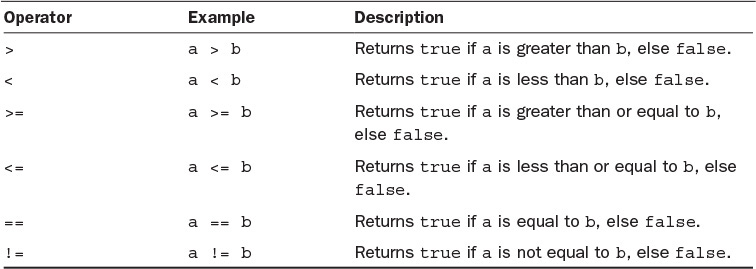TABLE 3.2 Comparison Operators

As with comparison operators, there are other advanced operators. We cannot discuss them all in this book, but we cover two more of them in Hour 10, “Learning About Structs and Classes.”

Note: Using Comparison Operators

Most often, comparison operators are used in conditional statements, such as an `if` block, or in loop statements, such as a `while` block. Examples would be `if a > b { print("a is greater than b" }`, or `while a < 100 { ++a }`. We cover conditionals and control flow in Hour 5, “Controlling Program Flow with Conditionals,” and loops in Hour 7, “Iterating Code with Loops.”

#### Range Operators

Range operators in Swift are denoted by two dots and a left angle bracket (`..<`) or three dots (`...`) depending on what type of range you need. There are two types of range operators: half-closed range operators and closed range operators.

A half-closed range operator uses two dots and a left angle bracket to indicate a range of integers from its left-hand operand up to but not including the right-hand operand. The expression `1..<5` defines a range of four integers, 1 through 4, but not including 5. You can also use variables or constants with range operators, such as `1..<a`. The half-closed operator is called as such because its range contains its initial value but not the final value.

A closed range operator uses three dots to indicate a range of integers from its left-hand operand up to and including the right-hand operand. The expression `-2...2` defines a range of five integers, from -2 to +2. The closed range operator can also use variables or constants as operands, such as `a...b`. The closed range operator is called closed range because the range is closed on each end by including both operands.

Caution: Ranges Must Progress in a Positive Direction

Ranges in Swift must progress in a positive direction. The starting index (left-hand operand) must be less than or equal to the ending index (right-hand operand). Attempting to progress in a negative direction will result in a compiler error if detected when coding or a run-time error and crash if detected while the app is running. You might want to validate that the left-hand operand is less than the right-hand operand if your operands are not known at compile time.

Both half-closed range operators and closed range operators are useful in iterative expressions. Closed range operators are good for ranges where you want both the first and last values of the range to be included. Half-closed range operators are especially good for zero-based lists such as arrays, where the index of the first element is 0, followed by 1, then 2, and so on. Arrays are discussed in more detail in Hour 4, “Working with Collection Types.” Consider the code in Listing 3.3—it illustrates the differences in the two range operators.

LISTING 3.3 Different Range Operators

`for a in 1...5 {    print(a)}//This closed range prints the following://1//2//3//4//5let arrayLength = 3for b in 0..<arrayLength {    print(b)}//This half-closed range prints the following://0//1//2`

#### Logical Operators

Video 3.3—Use Logical Operators

Swift provides three logical operators, AND (`&&`), OR(`||`), and NOT (`!`). These operators are called logical operators because they evaluate upon and return Boolean value types based on logic. The three logical operators compare the bits inside their given operands, perform the operation against them, and then return the result. These three operators are similar to those you would find in other languages, but we discuss them here if you’re not familiar.

##### Logical AND Operator

The logical AND operator, sometimes referred to as just AND, performs a logical AND operation against both operands and returns the result. Let’s illustrate how AND operates and comes up with a return value. Look at the truth table in Table 3.3.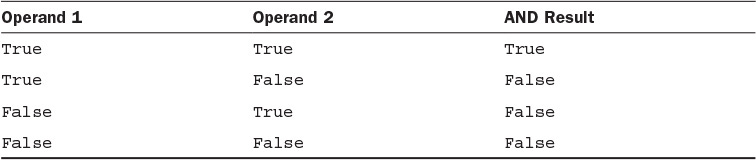TABLE 3.3 Logical AND Operator Truth Table

Let’s say you’re comparing two Boolean values, `a` and `b`, in the following code sample:

`let a = truelet b = falselet c = a && b`

What value does `c` have? You’re right; `c` is `false`. The AND operator is useful when every value in a certain set of conditions needs to be met first before executing an expression or set of expressions. For example, the following code snippet illustrates what use of the AND operator looks like if you were to try to get access to a device’s motion data:

`if CMPedometer.isStepCountingAvailable() && userAcceptedUseOfMotionProcessor {    /* it's now ok to get step data */} else {    print("cannot get step data")}`

If step counting is not available (known by the Boolean return value of `CMPedometer.isStepCountingAvailable()`) or if the user has explicitly stated it’s not okay for us to have access to the device’s motion coprocessor, then we cannot count the user’s steps.

##### Logical OR Operator

The logical OR operator is similar in syntax to the AND operator but behaves slightly differently. The OR operator evaluates two operands and decides whether either one is true or not, no matter which one, and then returns its result. The truth table in Table 3.4 details the OR operator’s behavior.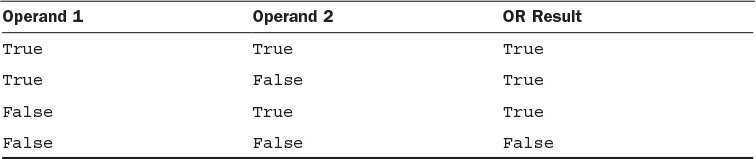TABLE 3.4 Logical OR Operator Truth Table

The OR operator returns true if either operand in the expression is true. Let’s take another look at a simple code snippet to illustrate OR:

`let a = truelet b = falselet c = a || b`

What is the value of `c`? Again, you’re right; the value is `true`. A more realistic example might look something like this:

`if userHasCellularConnectivity || userHasWifiConnectivity {    // assume user has an internet connection, start talking to web service} else {    // warn user to enable connectivity hardware}`

In the previous example, if either of the two operands (`userHasCellularConnectivity` or `userHasWifiConnectivity`) is true, then the first block of code executes. If neither operand is true, the second block of code executes. We cover conditionals with `if` statements in Hour 5.

##### Logical NOT Operator

The last logical operator, NOT, as mentioned earlier, simply inverts the value of a Boolean variable or constant. Although not a binary operator, NOT is listed here in the logical operators section for completeness. For example, see Table 3.5 for the truth table illustrating the values resulting from the NOT operator.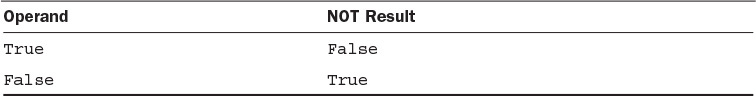TABLE 3.5 Logical NOT Operator Truth Table

##### Combining Logical Operators

Logical operators can be combined into larger expressions where multiple lines of logic may be required. The expression `a && b || !c` combines all three logical operators and can be read as “a and b must be true, or not c”. While not required, you can insert parentheses to explicitly state the order of operations you want in your code, such as `(a && b) || (!(c && d))`.

### Ternary Conditional Operators

Swift has only one ternary conditional operator, and it is written as short-hand for a longer expression that checks a particular case or condition and provides a value for the true branch and another value for the false branch. We cover the ternary conditional operator in greater detail in Hour 5, since it is a more succinct substitution for a longer conditional expression.

### Summary

In this hour, we covered a lot of material regarding operators. Most operators are straightforward, but if you don’t understand any, don’t worry; operators are used frequently throughout the book, and you will pick it up by repetition. Logical operators manipulate and/or combine Boolean values `true` and `false`. Unary operators act upon a single target, binary operators act upon two targets, and ternary operators compare a Boolean value to execute one of two expressions. Operators can be prefix, postfix, or infix, meaning they can be written immediately in front of an operand, immediately after an operand, or in between two operands, respectively. Most of these types of operators are familiar from other languages.

A set of operators in Swift that are new to some C and Objective-C programmers are range operators. These prove to be powerful for iterating over ranges of values, items in a list, and others. Range operators come in two types: half-closed range operators, which include the first but not the last values given, and closed range operators, which include the first and the last values given. We use these operators multiple times throughout this book.

In the next hour, we discuss collection types including `Arrays` and `Dictionaries`. `Arrays` and `Dictionaries` are crucial to many apps, in that they keep lists of multiple objects in memory to be used for things such as data sources, data retrieved from networks, or even to store preferences between app launches.

### Q&A

Q. Is it better to use postfix or prefix?

A. This depends on the needs of your calculations. There is no performance gain using one or the other; it purely depends on how you need to use them. For instance, `++a` and `a++` both increment `a`, but the former increments before returning its value, and the latter returns its value and then increments. Be careful of this distinction in your code.

Q. Why are there two range operators? Wouldn’t it be simpler to just use one?

A. Having two range operators allows you to express intent a lot more clearly in code. In the case of a zero-based list, you may have 10 items but the last item’s index is 9, so a half-closed range operator (`0..<count`, for instance) would be a better fit. If you know you have a finite number of times something should loop, a closed-range operator (`1...10`, for instance) clearly indicates a range of 1 to 10.

Q. What is a practical example of using remainder?

A. Imagine an app that shows a progress bar when loading. In this case, the progress is only checked occasionally (every nth time) throughout the loading loop, or when the count of the loop % n is equal to 0. This becomes clearer in later hours when we cover conditionals and control flow in Hour 5 and loops in Hour 7.

### Exercise

Create a new playground. Create two variables to hold two separate numbers, 1 and 10. Use different comparison operators to compare the two values. Next, display the numbers using both the half-closed and closed ranges.